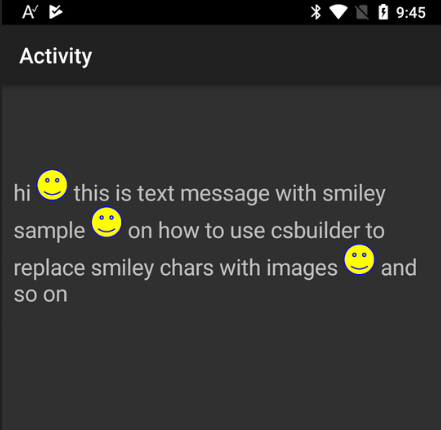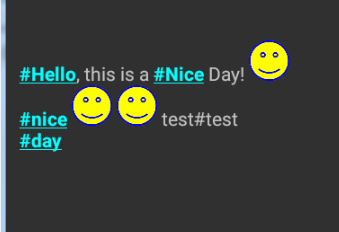# Android Code Snippet CSBuilder marking based on regex pattern

Discussion in 'Code Snippets' started by Erel, Aug 22, 2017.

1. This sub searches for matches and uses CSBuilder to mark the matches.Code:
`Sub MarkPattern(Input As String, Pattern As String, GroupNumber As Int) As CSBuilder   Dim cs As CSBuilder   cs.Initialize   Dim lastMatchEnd As Int = 0   Dim m As Matcher = Regex.Matcher(Pattern, Input)   Do While m.Find     Dim currentStart As Int = m.GetStart(GroupNumber)     cs.Append(Input.SubString2(lastMatchEnd, currentStart))     lastMatchEnd = m.GetEnd(GroupNumber)     'apply styling here     cs.Bold.Underline     cs.Color(0xFF03FFFF)     cs.Clickable("cs", m.Group(GroupNumber))     cs.Append(m.Group(GroupNumber))     cs.Pop.Pop.Pop.Pop 'number should match number of stylings set.   Loop   If lastMatchEnd < Input.Length Then cs.Append(Input.SubString(lastMatchEnd))   Return csEnd Sub`
Usage example:
Code:
`Sub Activity_Create(FirstTime As Boolean)   Activity.LoadLayout("1")   Dim s As String = \$"#Hello, this is a #Nice Day!#nice test#test#day"\$   Dim cs As CSBuilder = MarkPattern(s, "\B(#\w+)\b", 1)   Label1.Text = cs   cs.EnableClickEvents(Label1)End SubSub cs_Click (Tag As Object)   Log(\$"You have clicked on word: \${Tag}"\$)   Activity.Title = TagEnd Sub`

MarkusR, jmon, Pencil3 and 12 others like this.
2. Another example:Code:
`Sub Process_Globals   Private smileyBmp As BitmapEnd SubSub Globals   Private Label1 As LabelEnd SubSub Activity_Create(FirstTime As Boolean)   If FirstTime Then       smileyBmp = LoadBitmap(File.DirAssets, "smiley.png")   End If   Activity.LoadLayout("1")   Label1.Text = ReplaceSmilies("hi :) this is text message with smiley sample ;) on how to use csbuilder to replace smiley chars with images :-) and so on")   End SubSub ReplaceSmilies(s As String) As CSBuilder   Dim rb As RegexBuilder   rb.Initialize   For Each smiley As String In Array(":)", ";)", ":-)")       If rb.Pattern <> "" Then rb.AppendOr       rb.StartNonCapture.AppendEscaped(smiley).EndNonCapture   Next   Return MarkPattern(s, rb.Pattern, 0)End SubSub MarkPattern(Input As String, Pattern As String, GroupNumber As Int) As CSBuilder   Dim cs As CSBuilder   cs.Initialize   Dim lastMatchEnd As Int = 0   Dim m As Matcher = Regex.Matcher(Pattern, Input)   Do While m.Find       Dim currentStart As Int = m.GetStart(GroupNumber)       cs.Append(Input.SubString2(lastMatchEnd, currentStart))       lastMatchEnd = m.GetEnd(GroupNumber)       'apply styling here       Log(m.Group(GroupNumber))       cs.Image(smileyBmp, 30dip, 30dip, True)   Loop   If lastMatchEnd < Input.Length Then cs.Append(Input.SubString(lastMatchEnd))   Return csEnd Sub`
Notes:
1. The match itself is not appended as we replace it.
2. No need to call Pop after cs.Image

3. can each smile symbol have different image ?

4. Yes, of course. This is exactly why I left this line in the code:
Code:
`Log(m.Group(GroupNumber))`
For further discussion please start a new thread in the questions forum.

PassionDEV likes this.
5. can example 1 and example 2 work togehter?

6. Yes. The trick is to create a single pattern with all the capture groups:Code:
`Sub Activity_Create(FirstTime As Boolean)   If FirstTime Then       smileyBmp = LoadBitmap(File.DirAssets, "smiley.png")   End If   Activity.LoadLayout("1")   Dim s As String = \$"#Hello, this is a #Nice Day! :)#nice :) ;) test#test#day"\$   Dim rb As RegexBuilder   'The pattern includes 4 groups (1 - 4). One for the # words and three for the smilies.   rb.Initialize.StartCapture.Append("\B(?:#\w+)\b").EndCapture   For Each smiley As String In Array(":)", ";)", ":-)")       rb.AppendOr       rb.StartCapture.AppendEscaped(smiley).EndCapture   Next   Dim cs As CSBuilder = MarkPattern(s, rb.Pattern)   Label1.Text = cs   cs.EnableClickEvents(Label1)End SubSub cs_Click (Tag As Object)   Log(\$"You have clicked on word: \${Tag}"\$)   Activity.Title = TagEnd SubSub MarkPattern(Input As String, Pattern As String) As CSBuilder   Dim cs As CSBuilder   cs.Initialize   Dim lastMatchEnd As Int = 0   Dim m As Matcher = Regex.Matcher(Pattern, Input)   Do While m.Find       For GroupNumber = 1 To m.GroupCount - 1           If m.Group(GroupNumber) <> Null Then               Dim currentStart As Int = m.GetStart(GroupNumber)               cs.Append(Input.SubString2(lastMatchEnd, currentStart))               lastMatchEnd = m.GetEnd(GroupNumber)               If GroupNumber = 1 Then                   cs.Bold.Underline                   cs.Color(0xFF03FFFF)                   cs.Clickable("cs", m.Group(GroupNumber))                   cs.Append(m.Group(GroupNumber))                   cs.Pop.Pop.Pop.Pop               Else If GroupNumber >= 2 And GroupNumber <= 4 Then                   cs.Image(smileyBmp, 30dip, 30dip, True)               End If           End If       Next   Loop   If lastMatchEnd < Input.Length Then cs.Append(Input.SubString(lastMatchEnd))   Return csEnd Sub`

Alexander Stolte likes this.